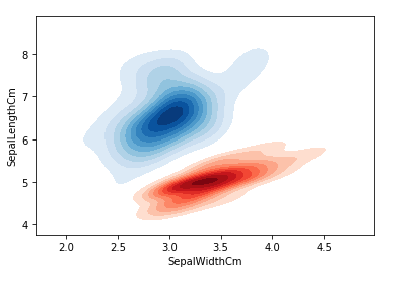﻿ Python Machine learning Scikit-learn: Create a kde plot of two shaded bivariate densities of Sepal Width and Sepal Length - w3resource# Python Scikit-learn: Create a kde plot of two shaded bivariate densities of Sepal Width and Sepal Length

## Python Machine learning Iris Visualization: Exercise-16 with Solution

Write a Python program using seaborne to create a kde (Kernel Density Estimate) plot of two shaded bivariate densities of Sepal Width and Sepal Length.

Sample Solution:

Python Code:

``````# Import necessary modules
import pandas as pd
import seaborn as sns
import matplotlib.pyplot as plt
#Drop id column
iris = iris.drop('Id',axis=1)
plt.title('Iris-setosa')
plt.xlabel('Petal Length Cm')
plt.ylabel('Petal Width Cm')
plt.show()
```
```

Output:

``````

Python Code Editor:

Have another way to solve this solution? Contribute your code (and comments) through Disqus.

Next: Write a Python program to find the correlation between variables of iris data. Also create a hitmap using Seaborn to present their relations.

What is the difficulty level of this exercise?

﻿